# RS Aggarwal Solutions for Class 7 Maths Chapter 9 Unitary Method

RS Aggarwal Solutions for Class 7 Maths Chapter 9 – Unitary Method are available here So that students can check for the solutions whenever they are facing difficulty while solving the question. These exercises are formulated by our expert tutors in order to assist you with your exam preparation to attain good marks in Maths. Students who wish to score good marks in Maths practise RS Aggarwal Class 7 Solutions

The main aim is to help students understand and crack these problems. We at BYJU’S have prepared the RS Aggarwal Solutions for Class 7 Maths Chapter 9 wherein problems are solved step by step with detailed explanations. Download pdf of Class 7 Chapter 9 in their respective links.

## Download PDF of RS Aggarwal Solutions for Class 7 Maths Chapter 9 – Unitary Method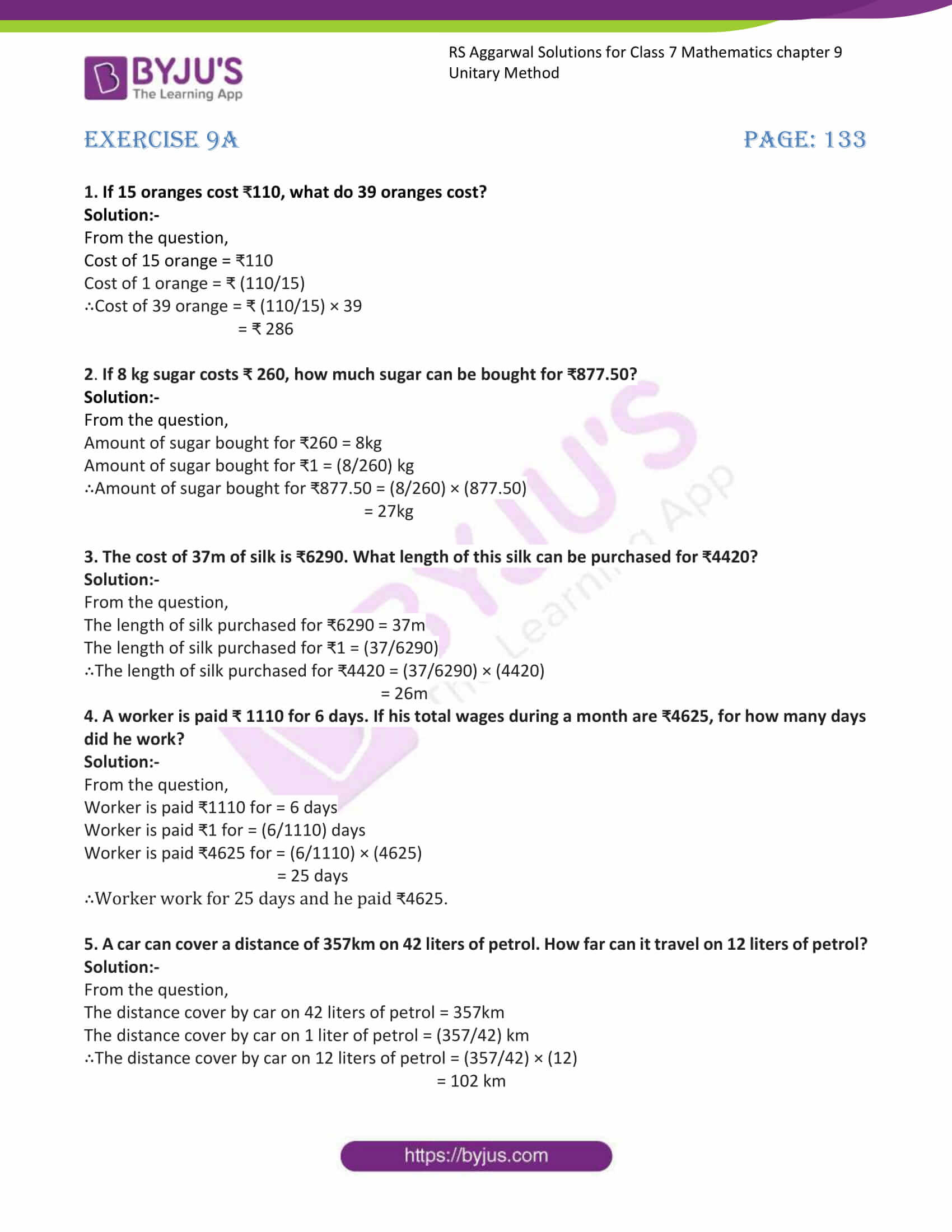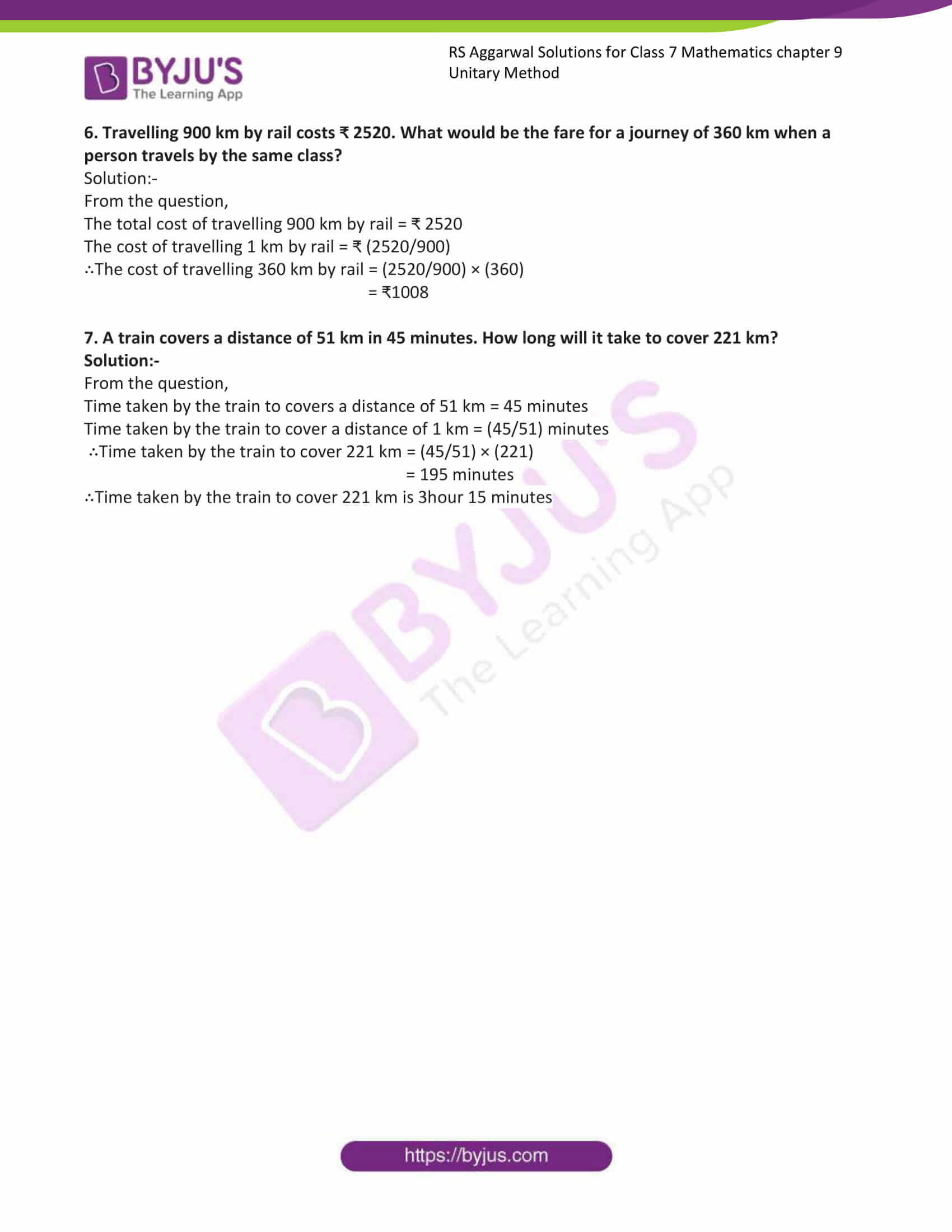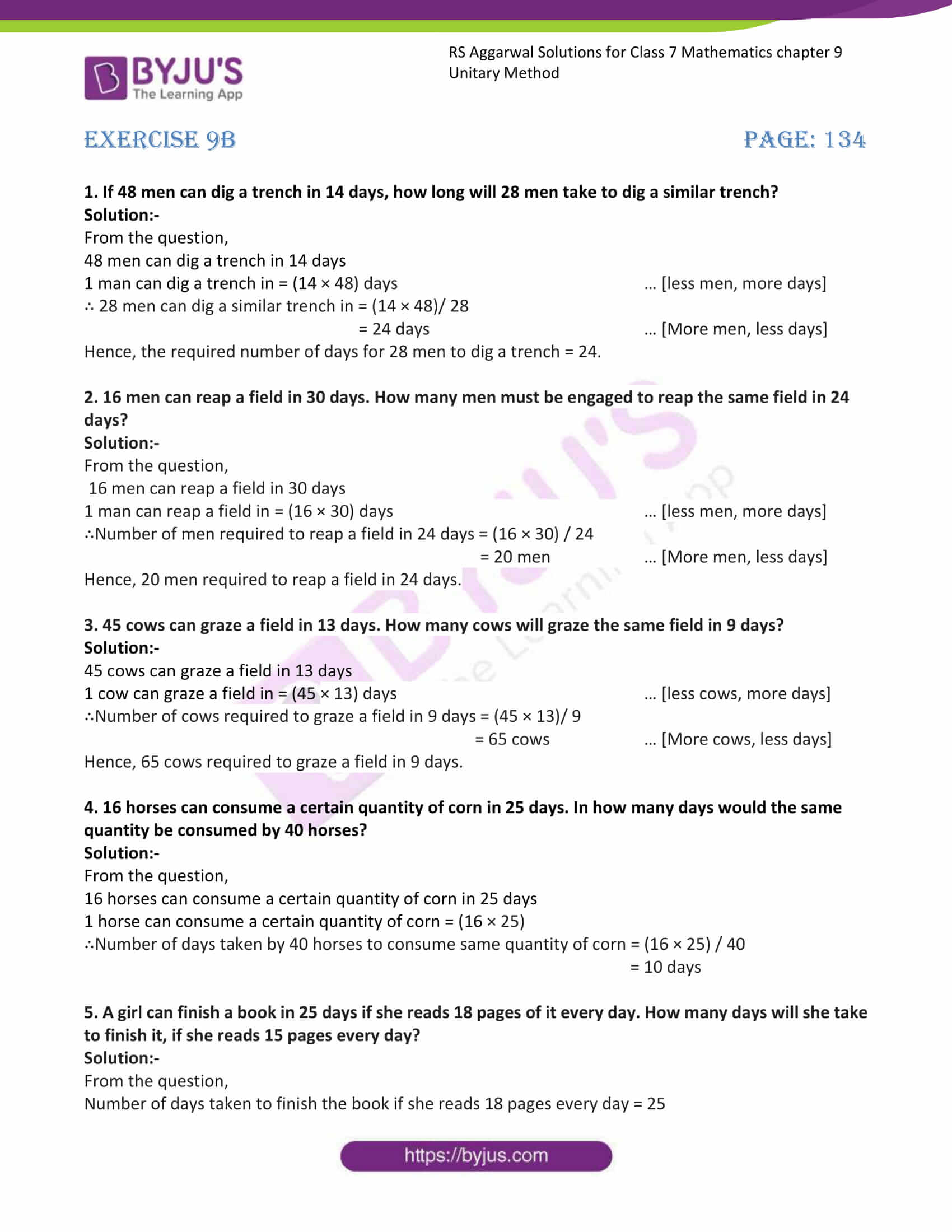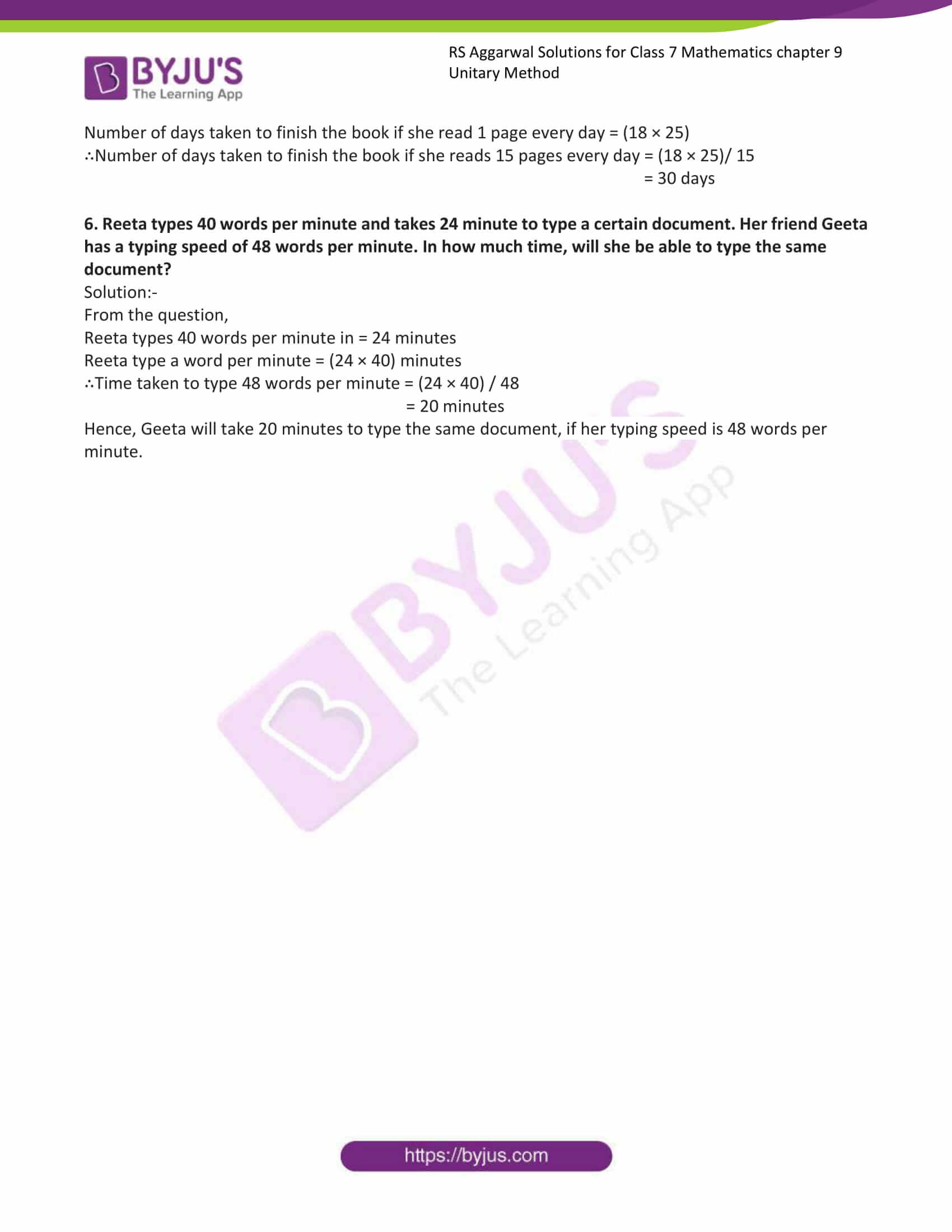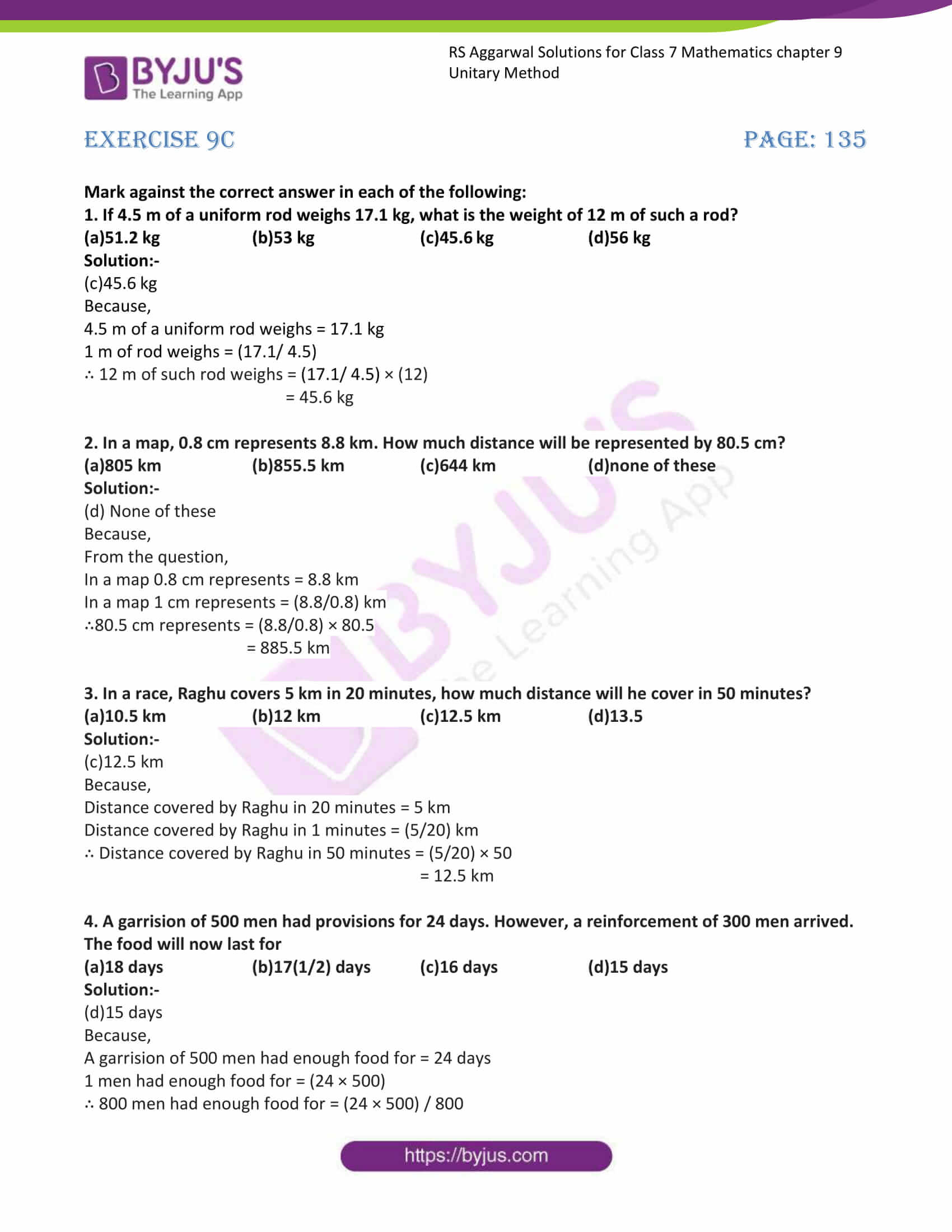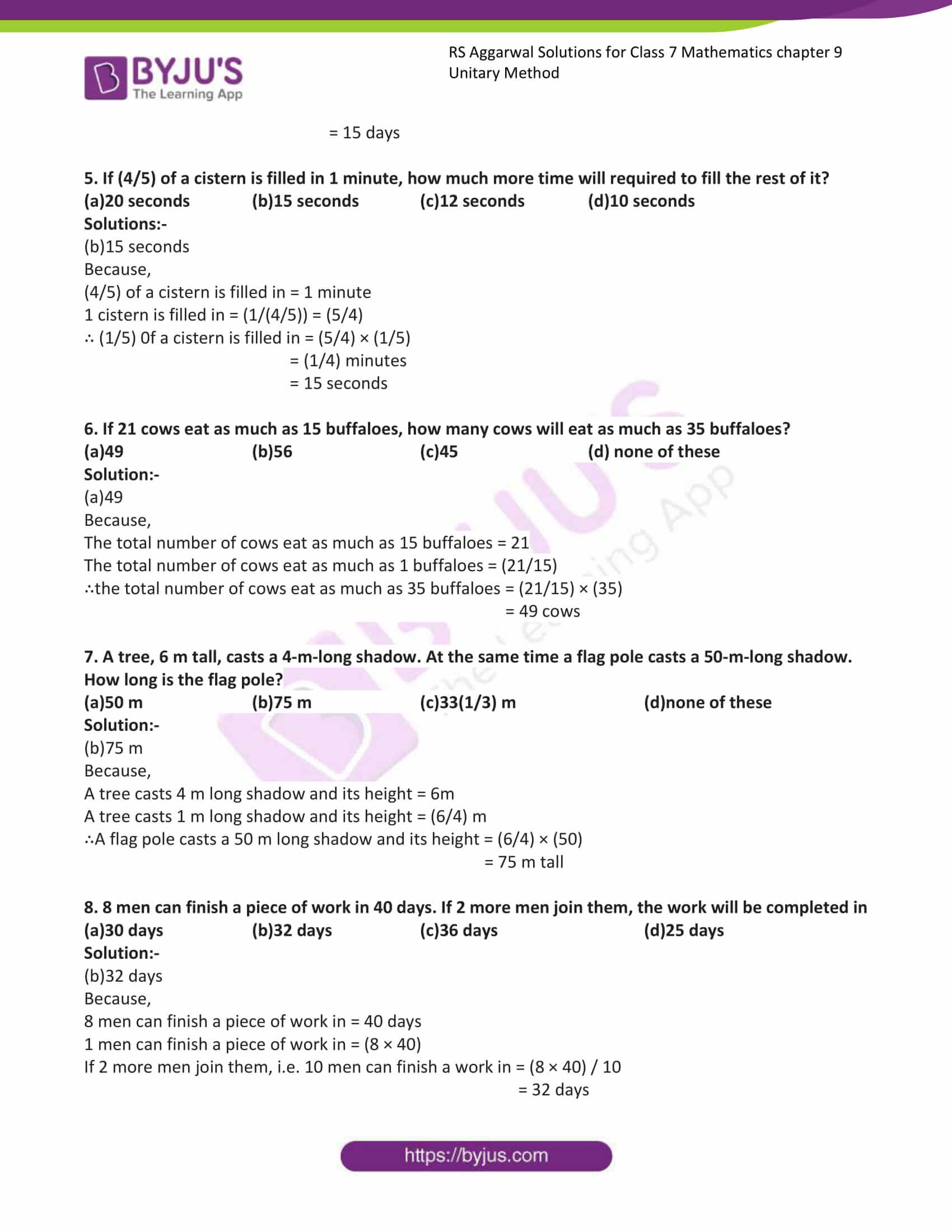Exercise 9A

Exercise 9B

Exercise 9C

## Exercise 9a

1. If 15 oranges cost ₹110, what do 39 oranges cost?

Solution:-

From the question,

Cost of 15 orange = ₹110

Cost of 1 orange = ₹ (110/15)

∴Cost of 39 orange = ₹ (110/15) × 39

= ₹ 286

2. If 8 kg sugar costs ₹ 260, how much sugar can be bought for ₹877.50?

Solution:-

From the question,

Amount of sugar bought for ₹260 = 8kg

Amount of sugar bought for ₹1 = (8/260) kg

∴Amount of sugar bought for ₹877.50 = (8/260) × (877.50)

= 27kg

3. The cost of 37m of silk is ₹6290. What length of this silk can be purchased for ₹4420?

Solution:-

From the question,

The length of silk purchased for ₹6290 = 37m

The length of silk purchased for ₹1 = (37/6290)

∴The length of silk purchased for ₹4420 = (37/6290) × (4420)

= 26m

4. A worker is paid ₹ 1110 for 6 days. If his total wages during a month are ₹4625, for how many days

did he work?

Solution:-

From the question,

Worker is paid ₹1110 for = 6 days

Worker is paid ₹1 for = (6/1110) days

Worker is paid ₹4625 for = (6/1110) × (4625)

= 25 days

∴Worker work for 25 days and he paid ₹4625.

5. A car can cover a distance of 357km on 42 liters of petrol. How far can it travel on 12 liters of petrol?

Solution:-

From the question,

The distance cover by car on 42 liters of petrol = 357km

The distance cover by car on 1 liter of petrol = (357/42) km

∴The distance cover by car on 12 liters of petrol = (357/42) × (12)

= 102 km

6. Travelling 900 km by rail costs ₹ 2520. What would be the fare for a journey of 360 km when a

person travels by the same class?

Solution:-

From the question,

The total cost of travelling 900 km by rail = ₹ 2520

The cost of travelling 1 km by rail = ₹ (2520/900)

∴The cost of travelling 360 km by rail = (2520/900) × (360)

= ₹1008

7. A train covers a distance of 51 km in 45 minutes. How long will it take to cover 221 km?

Solution:-

From the question,

Time taken by the train to covers a distance of 51 km = 45 minutes

Time taken by the train to cover a distance of 1 km = (45/51) minutes

∴Time taken by the train to cover 221 km = (45/51) × (221)

= 195 minutes

∴Time taken by the train to cover 221 km is 3hour 15 minutes

## Exercise 9B

1. If 48 men can dig a trench in 14 days, how long will 28 men take to dig a similar trench?

Solution:-

From the question,

48 men can dig a trench in 14 days

1 man can dig a trench in = (14 × 48) days … [less men, more days]

∴ 28 men can dig a similar trench in = (14 × 48)/ 28

= 24 days … [More men, less days]

Hence, the required number of days for 28 men to dig a trench = 24.

2. 16 men can reap a field in 30 days. How many men must be engaged to reap the same field in 24 days?

Solution:-

From the question,

16 men can reap a field in 30 days

1 man can reap a field in = (16 × 30) days … [less men, more days]

∴Number of men required to reap a field in 24 days = (16 × 30) / 24

= 20 men … [More men, less days]

Hence, 20 men required to reap a field in 24 days.

3. 45 cows can graze a field in 13 days. How many cows will graze the same field in 9 days?

Solution:-

45 cows can graze a field in 13 days

1 cow can graze a field in = (45 × 13) days … [less cows, more days]

∴Number of cows required to graze a field in 9 days = (45 × 13)/ 9

= 65 cows … [More cows, less days]

Hence, 65 cows required to graze a field in 9 days.

4. 16 horses can consume a certain quantity of corn in 25 days. In how many days would the same quantity be consumed by 40 horses?

Solution:-

From the question,

16 horses can consume a certain quantity of corn in 25 days

1 horse can consume a certain quantity of corn = (16 × 25)

∴Number of days taken by 40 horses to consume same quantity of corn = (16 × 25) / 40

= 10 days

5. A girl can finish a book in 25 days if she reads 18 pages of it every day. How many days will she take

to finish it, if she reads 15 pages every day?

Solution:-

From the question,

Number of days taken to finish the book if she reads 18 pages every day = 25

Number of days taken to finish the book if she read 1 page every day = (18 × 25)

∴Number of days taken to finish the book if she reads 15 pages every day = (18 × 25)/ 15

= 30 days

6. Reeta types 40 words per minute and takes 24 minute to type a certain document. Her friend Geeta

has a typing speed of 48 words per minute. In how much time, will she be able to type the same

document?

Solution:-

From the question,

Reeta types 40 words per minute in = 24 minutes

Reeta type a word per minute = (24 × 40) minutes

∴Time taken to type 48 words per minute = (24 × 40) / 48

= 20 minutes

Hence, Geeta will take 20 minutes to type the same document, if her typing speed is 48 words per

minute.

## Exercise 9C

Mark against the correct answer in each of the following:

1. If 4.5 m of a uniform rod weighs 17.1 kg, what is the weight of 12 m of such a rod?

(a)51.2 kg (b)53 kg (c)45.6 kg (d)56 kg

Solution:-

(c)45.6 kg

Because,

4.5 m of a uniform rod weighs = 17.1 kg

1 m of rod weighs = (17.1/ 4.5)

∴ 12 m of such rod weighs = (17.1/ 4.5) × (12)

= 45.6 kg

2. In a map, 0.8 cm represents 8.8 km. How much distance will be represented by 80.5 cm?

(a)805 km (b)855.5 km (c)644 km (d)none of these

Solution:-

(d) None of these

Because,

From the question,

In a map 0.8 cm represents = 8.8 km

In a map 1 cm represents = (8.8/0.8) km

∴80.5 cm represents = (8.8/0.8) × 80.5

= 885.5 km

3. In a race, Raghu covers 5 km in 20 minutes, how much distance will he cover in 50 minutes?

(a)10.5 km (b)12 km (c)12.5 km (d)13.5

Solution:-

(c)12.5 km

Because,

Distance covered by Raghu in 20 minutes = 5 km

Distance covered by Raghu in 1 minutes = (5/20) km

∴ Distance covered by Raghu in 50 minutes = (5/20) × 50

= 12.5 km

4. A garrision of 500 men had provisions for 24 days. However, a reinforcement of 300 men arrived.

The food will now last for

(a)18 days (b)17(1/2) days (c)16 days (d)15 days

Solution:-

(d)15 days

Because,

A garrision of 500 men had enough food for = 24 days

1 men had enough food for = (24 × 500)

∴ 800 men had enough food for = (24 × 500) / 800

= 15 days

5. If (4/5) of a cistern is filled in 1 minute, how much more time will required to fill the rest of it?

(a)20 seconds (b)15 seconds (c)12 seconds (d)10 seconds

Solutions:-

(b)15 seconds

Because,

(4/5) of a cistern is filled in = 1 minute

1 cistern is filled in = (1/(4/5)) = (5/4)

∴ (1/5) 0f a cistern is filled in = (5/4) × (1/5)

= (1/4) minutes

= 15 seconds

6. If 21 cows eat as much as 15 buffaloes, how many cows will eat as much as 35 buffaloes?

(a)49 (b)56 (c)45 (d) none of these

Solution:-

(a)49

Because,

The total number of cows eat as much as 15 buffaloes = 21

The total number of cows eat as much as 1 buffaloes = (21/15)

∴the total number of cows eat as much as 35 buffaloes = (21/15) × (35)

= 49 cows

7. A tree, 6 m tall, casts a 4-m-long shadow. At the same time a flag pole casts a 50-m-long shadow.

How long is the flag pole?

(a)50 m (b)75 m (c)33(1/3) m (d)none of these

Solution:-

(b)75 m

Because,

A tree casts 4 m long shadow and its height = 6m

A tree casts 1 m long shadow and its height = (6/4) m

∴A flag pole casts a 50 m long shadow and its height = (6/4) × (50)

= 75 m tall

8. 8 men can finish a piece of work in 40 days. If 2 more men join them, the work will be completed in

(a)30 days (b)32 days (c)36 days (d)25 days

Solution:-

(b)32 days

Because,

8 men can finish a piece of work in = 40 days

1 men can finish a piece of work in = (8 × 40)

If 2 more men join them, i.e. 10 men can finish a work in = (8 × 40) / 10

= 32 days

## RS Aggarwal Solutions for Class 7 Maths Chapter 9 – Unitary Method

Chapter 9 – Unitary Method contains 3 exercises and the RS Aggarwal Solutions available on this page provide solutions to the questions present in the exercises. Now, let us have a look at some of the concepts discussed in this chapter.

• Direct Variation
• Inverse Variation

### Chapter Brief of RS Aggarwal Solutions for Class 7 Maths Chapter 9 – Unitary Method

RS Aggarwal Solutions for Class 7 Maths Chapter 9 – Unitary Method is a technique for solving a problem by first finding the value of a single unit, and then finding the necessary value by multiplying the single unit value. In essence, this method is used to find the value of a unit from the value of a multiple, and hence the value of a multiple. The solutions solved in such a way that students will understand clearly.